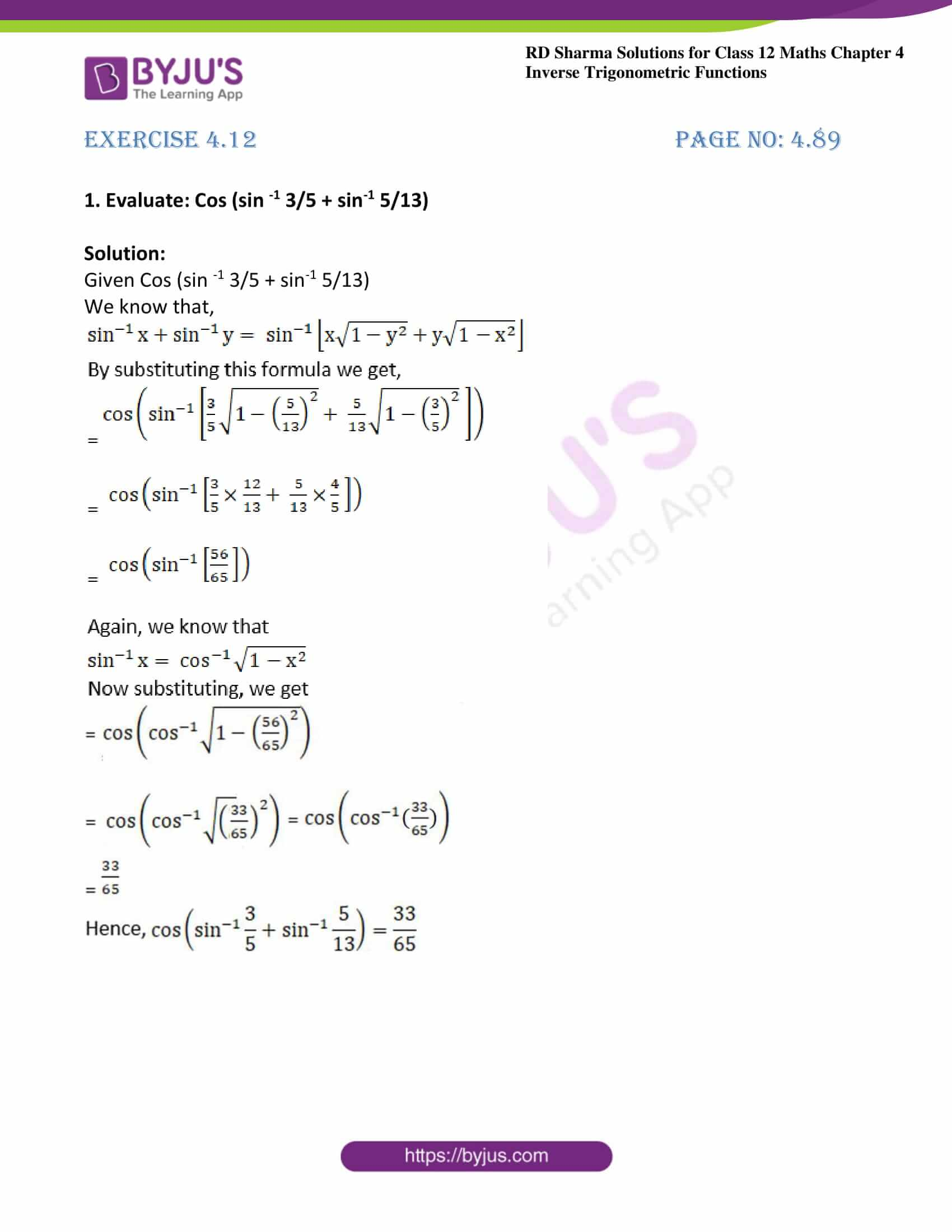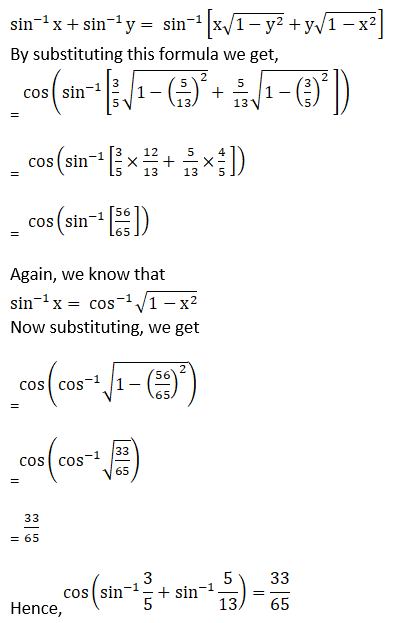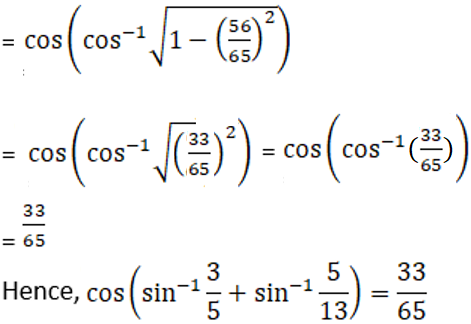# RD Sharma Solutions For Class 12 Maths Exercise 4.12 Chapter 4 Inverse Trigonometric Functions

RD Sharma Solutions for Class 12 Maths Exercise 4.12 Chapter 4 Inverse Trigonometric Functions is provided here. In this exercise, we shall discuss the properties of inverse trigonometric functions. The primary objective is to help students improve their problem-solving abilities, which in turn, helps in boosting their confidence level to achieve high marks in their exams.

Students can refer and download RD Sharma Solutions for Class 12 Maths Exercise 4.12 Chapter 4 Inverse Trigonometric Functions. These RD Sharma Solutions are formulated by BYJU’S expert team in Maths.

## Download the PDF of RD Sharma Solutions For Class 12 Chapter 4 – Inverse Trigonometric Functions Exercise 4.12### Exercise 4.12 Page No: 4.89

1. Evaluate: Cos (sin -1 3/5 + sin-1 5/13)

Solution:

Given Cos (sin -1 3/5 + sin-1 5/13)

We know that,### Access other exercises of RD Sharma Solutions For Class 12 Chapter 4 – Inverse Trigonometric Functions

Exercise 4.1 Solutions

Exercise 4.2 Solutions

Exercise 4.3 Solutions

Exercise 4.4 Solutions

Exercise 4.5 Solutions

Exercise 4.6 Solutions

Exercise 4.7 Solutions

Exercise 4.8 Solutions

Exercise 4.9 Solutions

Exercise 4.10 Solutions

Exercise 4.11 Solutions

Exercise 4.13 Solutions

Exercise 4.14 Solutions